# Empty and full

An empty can has a mass of 1/6 lb. When it is filled with sand, it has a mass of 7/12 lb. Find the mass of the sand in the can?

s =  0.4167 lb

### Step-by-step explanation: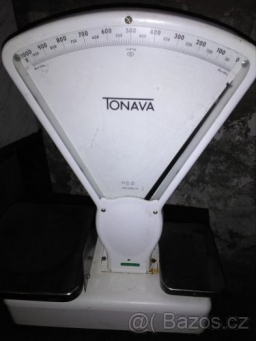Did you find an error or inaccuracy? Feel free to write us. Thank you!Tips to related online calculators
Need help to calculate sum, simplify or multiply fractions? Try our fraction calculator.

## Related math problems and questions:

• Baxter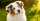Baxter ate 1/12 of a box of dog food. Now the box is 3/4 full. What fraction of the full of a full box was there before Baxter?
• BarrelsPeter has 42 barrels. One-sixth of them are filled with lemonade, one third is filled with wine and half is empty. Calculate how many barrels are with each filling.
• Three friendsJohn, Peter, and Pablo each carried a 24 liters bucket full of water down the hill. After they reached the bottom, John's bucket was only 3/4 full, Peter's bucket was 2/3 full, and Pablo's was 1/6 full. How much liters of water did they spill altogether o
• Bag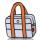Nelly found interesting point. An empty bag weighs 4 kg less than full. And empty bag is 5 times easier than full. How many kg weigh things in this bag?
• Sand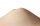How much m ^ 3 of sand can be loaded on a car with a load capacity of 5 t? The sand density is 1600 kg/m3 .
• Packed sand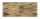We need 5 kilograms of sand. When he went to the store, the bags of sand only stated the number of grams on the package. How many grams should he look for on the package of sand? (1 kilogram= 1,000 grams) A. 5,000 grams B. 50,000 grams C. 50 grams D. 500
• SandThe maximum weight of the car is 5000 kg. 10 m3 of sand must be transferred. How many times does a car have to go? (density of sand is 1500 kg/m3)
• A 14.5-gallonA 14.5-gallon gasoline tank is 3/4 full. How many gallons will it take to fill the tank? Write your answer as a mixed number.
• Find the 24Find the difference between 2/7 and 1/21
• Excavator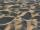The excavator bucket capacity is 0.5 m3. Determine the mass of sand that the excavator picks up. The sand density is 1700 kg/m3.
• Hotel 4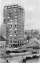A 360 room hotel has 1/3 of its rooms occupied at present . How many rooms are empty?
• A fullA full bag of dog food weights 5 1/2 pounds. How much dog food do you have if there are 3 3/4 bags in the cupboard?
• Kittens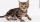Sometimes Grace has kittens. Each kitten has 1/3 as much cat food as a full-grown cat. How many kittens can Grace feed with the 1.47 pounds? Solve on paper. Then check your work. 1 full-grown cat need 0.25 pound of food.The fruit basket is five times heavier than the empty basket. Filled is 20kg heavier than empty. Determine the weight of the fruit in the basket.Dana helped her dad build a sandbox for her younger sister. The sandbox is shaped like a rectangular prism that is 4 1/2 feet long and 4 feet wide. Dana used bags of sand to fill the sandbox 1/2 of a foot deep. Each bag contained 1/2 of a cubic foot of sa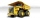The truck with a load capacity of 7 tons has a storage area of 4.5 m and 2.1 m. The weight of 1 m3 of wet sand is 2,000 kg. How high can we load the sand so that the load capacity of the car is not exceeded?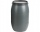In the backyard is an empty barrel with a volume of 2 hl. How many liters are missing at the edge, when 53 liters flowed into it for 1 day and 68 liters of water for 2 days?